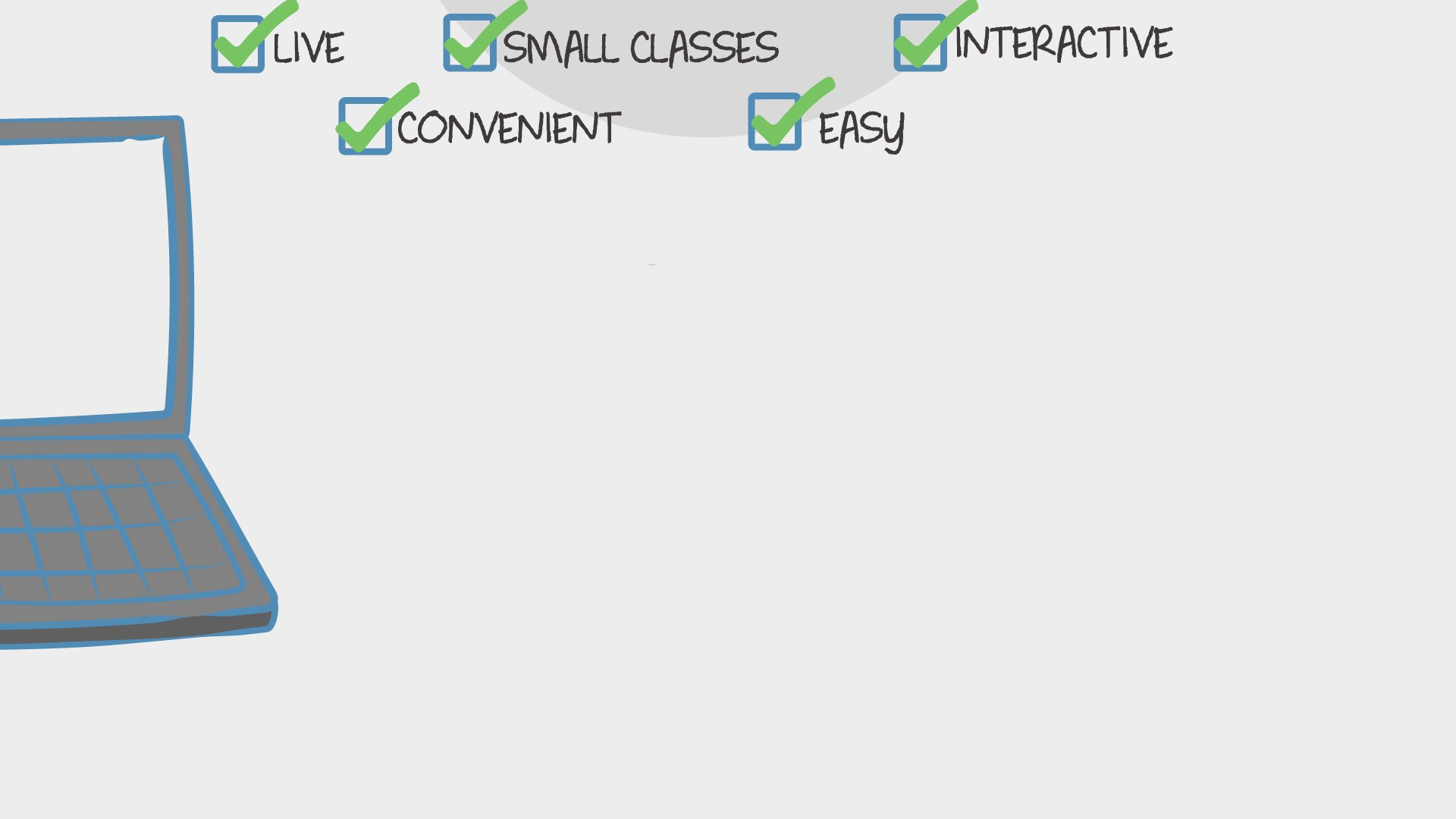Find Classes
Teach

Math

# 2nd Grade Math (weeks 1-8)

In this 8 week long class, we will meet all the common core math standards for the 1st quarter of 2nd grade.547 total reviews for this teacher
4 reviews for this class
Completed by 25 learners
There are no upcoming classes.25 minutes
per class
3x per week
over 8 weeks
6-9
year olds
2-6
learners per class
per learner - per class

### How does a “Multi-Day” course work?

Meets multiple times at scheduled times
Live video chats, recorded and monitored for safety and quality
Discussions via classroom forum and private messages with the teacher
Great for engaging projects and interacting with diverse classmates from other states and countries### How Outschool Works### There are no open spots for this class.

You can request another time or scroll down to find more classes like this.

## Description

### Class Experience

```Common Core Math Standards that we will hit in this class.

Represent and solve problems involving addition and subtraction.
37 2.OA.1  Use addition and subtraction within 100 to solve one- and two-step word problems involving situations of adding to, taking from, putting together, taking apart, and comparing, with unknowns in all positions, e.g., by using drawings and equations with a symbol for the unknown number to represent the problem.

2.OA.2  Fluently add and subtract within 20 using mental strategies.

By end of grade 2, know from memory all sums of two one-digit numbers.
Use place value understanding and properties of operations to add and subtract.
2.NBT.5  Fluently add and subtract within 100 using strategies based on place value, properties of operations, and/or the relationship between addition and subtraction.

Measure and estimate lengths in standard units.
2.MD.1  Measure the length of an object by selecting and using appropriate tools such as rulers, yardsticks, meter sticks, and measuring tapes.
2.MD.2  Measure the length of an object twice, using length units of different lengths for the two measurements; describe how the two measurements relate to the size of the unit chosen. 2.MD.3  Estimate lengths using units of inches, feet, centimeters, and meters. 2.MD.4  Measure to determine how much longer one object is than another, expressing the length difference in terms of a standard length unit.

Relate addition and subtraction to length.
2.MD.5  Use addition and subtraction within 100 to solve word problems involving lengths that are given in the same units, e.g., by using drawings (such as drawings of rulers) and equations with a symbol for the unknown number to represent the problem.
2.MD.6  Represent whole numbers as lengths from 0 on a number line diagram with equally spaced points corresponding to the numbers 0, 1, 2, …, and represent whole-number sums and differences within 100 on a number line diagram.

Understand place value.
2.NBT.1  Understand that the three digits of a three-digit number represent amounts of hundreds, tens and ones; e.g., 706 equals 7 hundreds, 0 tens, and 6 ones. Understand the following as special cases:  a. 100 can be thought of as a bundle of ten tens – called a “hundred.” b. The numbers 100, 200, 300, 400, 500, 600, 700, 800, 900 refer to one, two, three, four, five, six, seven, eight, or nine hundreds (and 0 tens and 0 ones).
2.NBT.2  Count within 1000; skip-count by 5s42, 10s, and 100s.
2.NBT.3  Read and write numbers to 1000 using base-ten numerals, number names, and expanded form.
2.NBT.4  Compare two three-digit numbers based on meanings of the hundreds, tens, and ones digits, using >, =, and < symbols to record the results of comparisons

```
`I taught 2nd grade math for 7 years. I have a degree in Elementary Education with an emphasis in math and social sciences.`
`Students will have optional homework to complete after certain classes. This will help me, the parents, and the students see if any more instruction is needed for those skills. It will also provide extra practice for the students.`
`Students will need paper and a pencil for each class. A whiteboard and marker work also. Any other materials will be attached for parents to print out.`
`Learners will not need to use any apps or websites beyond the standard Outschool tools.`
`I will use homework and observations to see how each student is progressing. Throughout the semester I will discuss the students progress with the parents.`
`1 hour 15 minutes per week in class, and maybe some time outside of class.`
`We will be using common core standards.`

## Teacher

`Hi, my name is Madison. I have a Bachelor's of Science Degree in Elementary Education with an emphasis in Social Science and Math. I have a 5 year old son and a two year old daughter. We love to play outside and create art with the things we find... `